# How to calculate number of days in a month or a year in Excel?

The number of days in a leap year is 366, whereas the number of days in a common year is 365. As is general knowledge, there are both leap years and common years. To determine, from a given date, the total number of days that comprise a month or a year.

When you have a list of dates organised in a column, you can use the formulas given in this tutorial to compute the number of days in each month. Let’s understand step by step with an example.

## Step 1

In the first step, Let’s assume we have a sample data for calculate the number of days in a month as shown in the below screenshot for the same.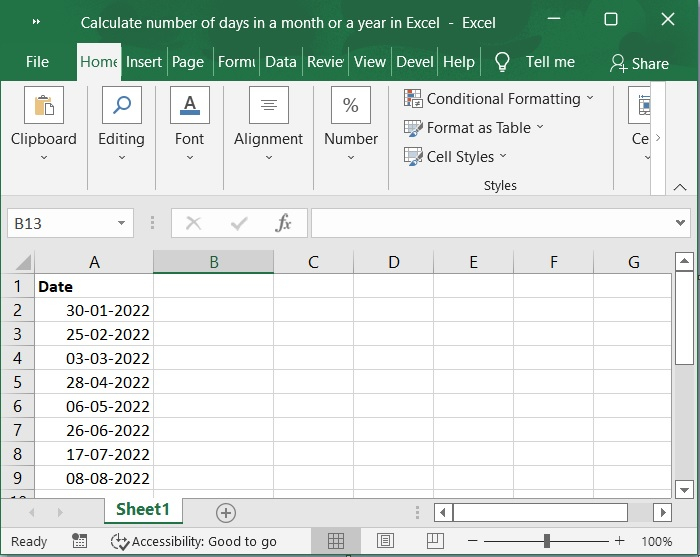## Step 2

We have a list of dates in cell A2:A9 and you want to calculate the number of days in a month. So, this formula can be entered into a blank cell in column B. Please check out the screenshot below for same.

=DAY(DATE(YEAR(A2),MONTH(A2)+1,1)-1)
=DAY(EOMONTH(A2,0))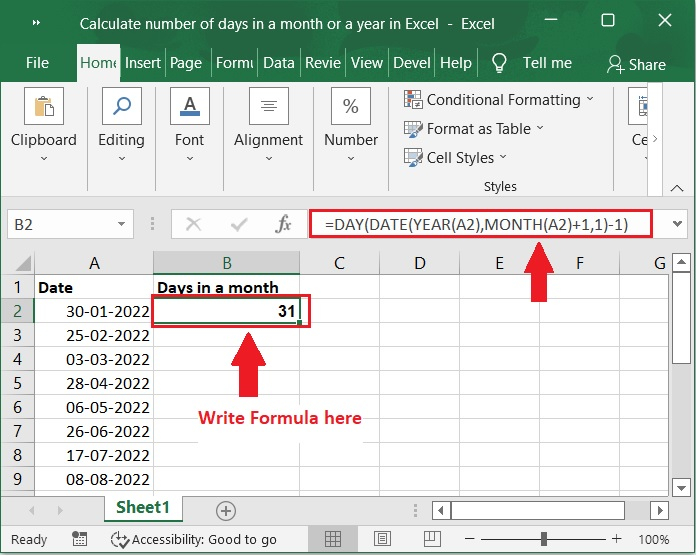## Step 3

Then, to calculate the number of days in a certain month, drag the fill handle down until it is over the cells that you want to use.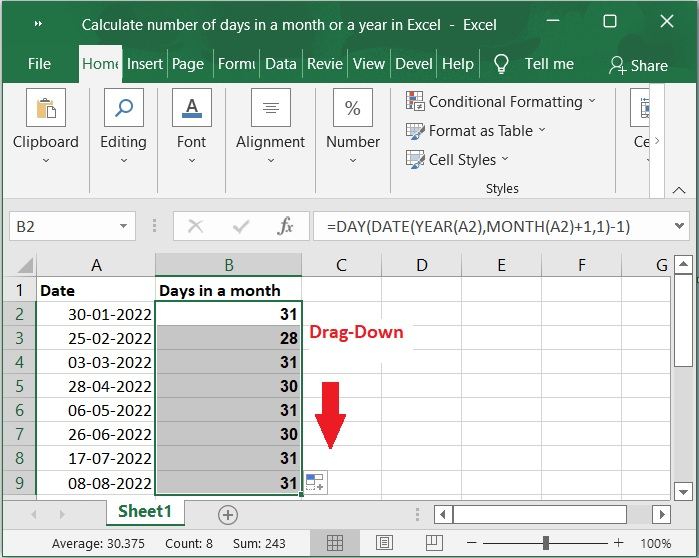Important Note − In the following formulas, cell A2 is the date from which you want to find out how many days are in a month.

To determine how many days there are in a year, please follow these instructions.

## Step 4

We have a list of dates in cell A2:A9 and you want to calculate the number of days in a Year. So, this formula can be entered into a blank cell in column "C".

=DATE(YEAR(A2),12,31)-DATE(YEAR(A2),1,1)+1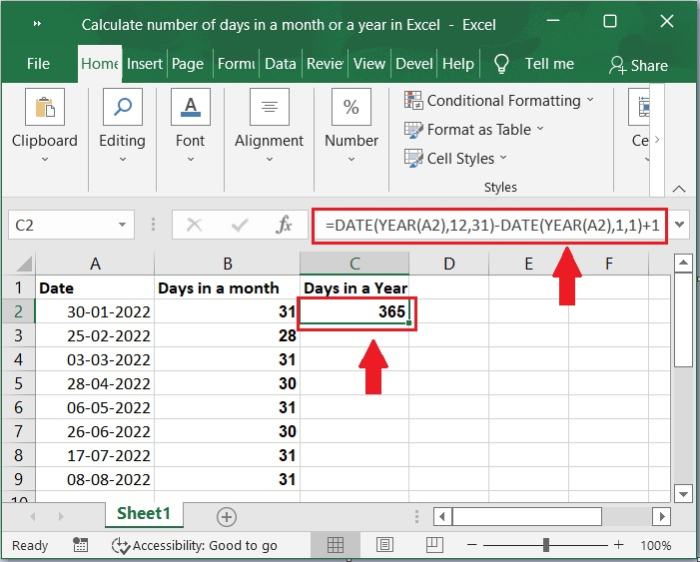## Step 5

Then, to calculate the number of days in a certain Year, drag the fill handle down until it is over the cells that you want to use. Please refer to the screenshot below for the same.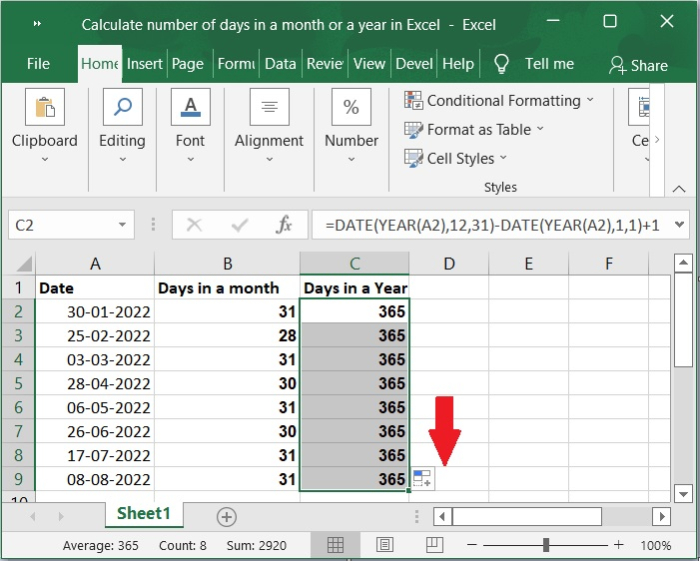Important Note − The following formula can be used to determine the number of days in the provided years if just the corresponding years are included in the column.

=365+IF(MOD(A2,4),0,1)


## Conclusion

In this tutorial, we explained with a simple example how you can calculate the number of days in a month and number of days in a year by using formulas in Excel.

Updated on: 03-Feb-2023

583 Views